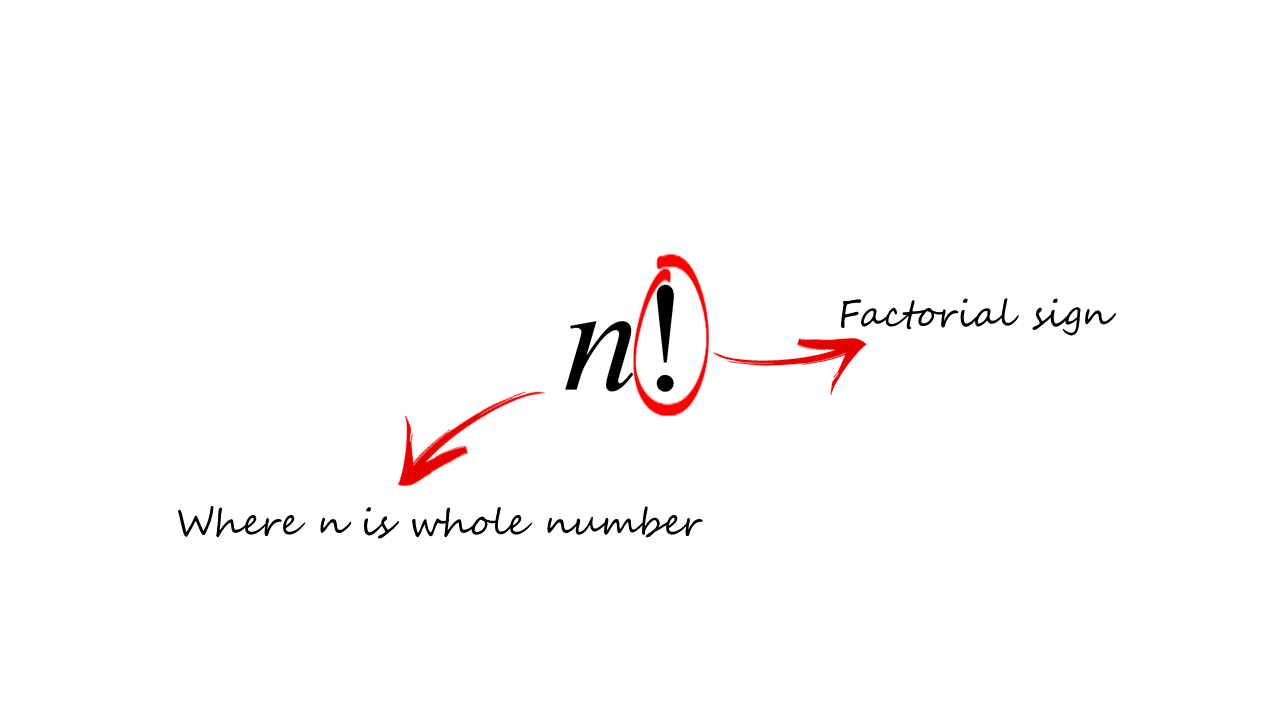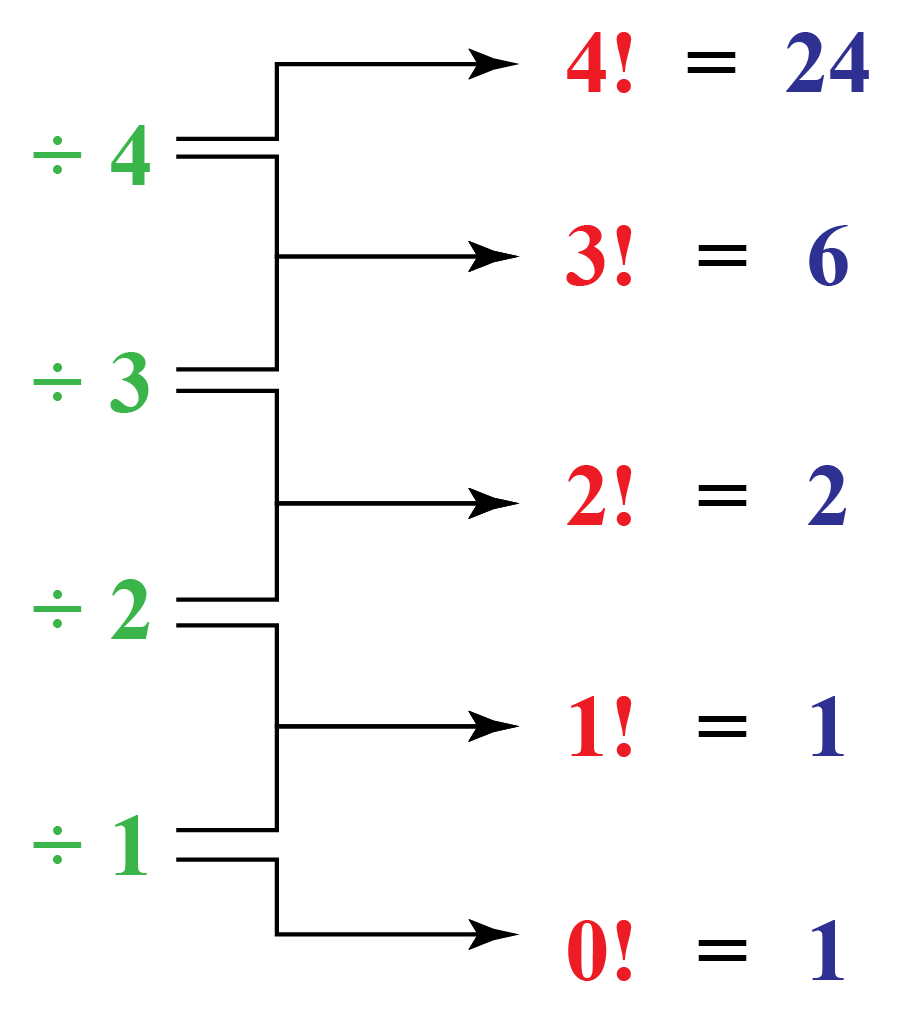# Factorial

In this mini-lesson, we will learn concepts related to factorial. They include n+1 factorial, the factorial of 10, the factorial of 0, combinations, and permutations.

Before we start, let's see how it all began.

In the year 1677, Fabian Stedman, a British author, defined factorials as an equivalent of change ringing. Change ringing was a part of the musical performance where the musicians would ring multiple tuned bells.

And it was in the year 1808, when a mathematician from France, Christian Kramp, came up with the symbol for factorial: n!

You will be surprised to know that the study of factorials is at the root of several topics in mathematics, such as number theory, algebra, geometry, probability, statistics, graph theory, and discrete mathematics, etc.

Look at the factorial calculator below.

Just substitute the value of $$n$$ to get its factorial value.

Thinking about how to calculate the factorial of a number? Let's learn.

## Lesson Plan

 1 What Is Factorial? 2 Solved Examples on Factorial 3 Interactive Questions on Factorial

## What Is Factorial?

A factorial of a number is the function which multiples the number by every natural number below it. Symbolically the factorial can be represented as "!"

So, n factorial is the product of first n natural numbers, represented as n!So n! or "$$n$$ factorial" means:

n! = 1. 2. 3…………………………………n

=  Product of the first n positive integers

=  n(n-1)(n-2)…………………….(3)(2)(1)

For example,

4 factorial, that is, 4! can be written as:

4! = $$4 \times 3 \times 2 \times 1$$ = 24

Let us look at a few numbers on the table.

 n n! 1 1 1 1 2 $$2 \times 1$$ = $$2 \times 1!$$ = 2 3 $$3 \times 2 \times 1$$ = $$3 \times 2!$$ = 6 4 $$4 \times 3 \times 2 \times 1$$ = $$4 \times 3!$$ = 24 5 $$5 \times 4 \times 3 \times 2 \times 1$$ = $$5 \times 4!$$ = 120 6 etc... etc...
• To work out for 6! multiply 120 by 6, to get 720

• To work out for 7! multiply 720 by 7, to get 5040

## Formula for n Factorial

The factorial formula or formula for n factorial is given by,

 $n! = n \times (n - 1)!$

This means that the factorial of any number is that number times the factorial of that number minus 1

So, $$8! = 8 \times 7!$$......

And $$9! = 9 \times 8!$$......

The factorial of 10 will be  $$10! = 10 \times 9!$$......

Like this if we have (n+1) factorial then it can be written as, $$(n+1)! = (n+1) \times n!$$.

### What Is 0!

Zero factorial or Factorial of 0 is interesting, and its value is equal to 1, i.e.  0! = 1Let us see that how this works:

$$\begin{array}{l} 1! = 1 \\ 2! = 2 \times 1 = 2 \\ 3! = 3 \times 2 \times 1 = 3 \times 2! = 6 \\ 4! = 4 \times 3 \times 2 \times 1 = 4 \times 3! = 24 \\ 5! = 5 \times 4 \times 3 \times 2 \times 1 = 5 \times 4! = 120 \\ \end{array}$$

Let’s go to the basic formula of factorial $$n! = n \times (n - 1)!$$.

How to find 4! What you do is $$\dfrac{{5!}}{5}$$.

Now, let’s look at the pattern:Can we have factorials for numbers like −1, −2, etc?

Let's start with 3! = $$3 \times 2 \times 1 = 6$$

$$\begin{array}{l} {\rm{\text{Let's start with } \ 3!= 3 \times 2 \times 1 \\ = 6\: \text{and go down }:}} \\ {\rm{2! = 3! / 3 = 6 / 3 = 2}}\\ {\rm{1! = 2! / 2 = 2 / 2 = 1}} \\ {\rm{0! = 1! / 1 = 1 / 1 = 1 }}\\ \left( {{\rm{ - 1}}} \right){\rm{! = 0! / 0 = 1 / 0 \\= {\text{ dividing by zero is undefined}}}} \\ \end{array}$$

And from here on down all integer factorials are undefined.

So, negative integer factorials are undefined.

## Where Is Factorial Used?

One area where they are commonly used is in permutations & combinations.

A permutation is an arrangement of outcomes in which the order does matter.

It can be calculated as

 nPr = $$\dfrac{{n!}}{{(n - r)!}}$$

A combination is a grouping of outcomes in which the order does not matter.

It can be calculated as

 nCr = $$\dfrac{{n!}}{{r!(n - r)!}}$$

Let us understand by taking examples,

Example 1

In a group of 10 people, Rs.200, Rs.100, and Rs.50 prizes are to be given. How many ways can the prizes be distributed?

Solution:

This is permutation because here the order matters — who get Rs.20 or  Rs.10 or Rs.5

It can be calculated as $$^{{\rm{1}}0}{{\rm{P}}_{\rm{3}}}$$ ways.

$$^{{\rm{1}}0}{{\rm{P}}_{\rm{3}}} = \dfrac{{10!}}{{(10 - 3)!}} = \dfrac{{10!}}{{7!}} \\= \dfrac{{10 \times 9 \times 8 \times 7!}}{{7!}} = 720$$ ways.

Example 2

In a group of 10 people, three Rs.50 prizes are to be given. How many ways can the prizes be distributed?

Solution:

This is a combination because here the order does not matter.

It can be calculated as, $$^{{\rm{1}}0}{{\rm{C}}_{\rm{3}}}$$ ways.

$$^{{\rm{1}}0}{{\rm{C}}_{\rm{3}}} = \dfrac{{10!}}{{3!(10 - 3)!}} = \dfrac{{10!}}{{3!7!}} \\= \dfrac{{10 \times 9 \times 8 \times 7!}}{{3 \times 2 \times 1 \times 7!}} = 120$$ ways.

## How Do You Calculate Factorial?

The factorial of n is denoted by n! and calculated by the integer numbers from 1 to n.

The formula for n factorial is  $$n! = n \times (n - 1)!$$.

Example

If $$8!$$ is 40,320 then what is 9!?

Solution

$$9! = 9 \times 8! = 9 \times 40,320 = 362,880$$

Look at the factorial calculator below to calculate combination.

Just drag the slider to change the value of n and r and see the value of n factorial and its combination.

A small factorial table:

 n n! 1 1 2 2 3 6 4 24 5 120 6 720 7 5040 8 40,320 9 362,880 10 3,628,800 11 39,916,800 12 479,001,600 13 6,227,020,800 14 8,717,8291,200 15 1,307,674,368,000

As you can see, it gets big quite drastically.

 Example 1

Evaluate the expression $$\dfrac{{10!}}{{4! \times 6!}}$$

Solution

$\begin{array}{l} \dfrac{{10!}}{{4! \times 6!}} &= \dfrac{{10 \times 9 \times 8 \times 7 \times 6!}}{{4! \times 6!}} \\[0.2cm] &= \dfrac{{10 \times 9 \times 8 \times 7}}{{4 \times 3 \times 2 \times 1}} \\[0.2cm] &= 210 \\ \end{array}$

Therefore, the value of expression $$\dfrac{{10!}}{{4! \times 6!}}$$ is 210

 $$\therefore$$ The value is 210
 Example 2

Find the value of  $$5!(6 - 3)!$$

Solution

$\begin{array}{l} 5!(6{\rm{ }} - {\rm{ }}3)!{\rm{ }} &= {\rm{ }}5! \times 2! \\[0.2cm] {\rm{ }} &= (5 \times 4 \times 3 \times 2 \times 1)(2 \times 1) \\[0.2cm] {\rm{ }} &= 120 \times 2 \\[0.2cm] {\rm{ }} &= 240 \\ \end{array}$

Therefore, the value of  $$5!(6 - 3)!$$ is 240

 $$\therefore$$ The value is 240
 Example 3

How many 5-digit numbers can be formed using the digits 1, 2, 5, 7 and 8 in each of which no digit is repeated?

Solution

The given 5 digits (1, 2, 5, 7 and 8) should be arranged among themselves in order to get 5-digit numbers.

The number of ways for doing this can be done using the factorial.

$5! = 5 \times 4 \times 3 \times 2 \times 1 = 120$

 $$\therefore$$  the required number of 5-digit numbers is, 120
 Example 4

How many ways can sprinters come first, second, and third in a row with 10 volunteers?Solution

We need to count all the possibilities in which

we can select 3 different runners in different orders. This can be selected in $$^{{\rm{1}}0}{{\rm{P}}_{\rm{3}}}$$ ways.

$^{{\rm{1}}0}{{\rm{P}}_{\rm{3}}} = \dfrac{{10!}}{{7!}} = 720$

 $$\therefore$$ Sprinters can come first, second, and third in 720 ways.
 Example 5

In how many ways can eight people line up from left to right for a group photo?Solution

\begin{align}&\text{Number of ways 8 people line up}\\[0.2cm] &= 8! \\[0.2cm] &= 8 \times 7 \times 6 \times 5 \times 4 \times 3 \times 2 \times 1 \\[0.2cm] &= 40,320 \\ \end{align}

 $$\therefore$$ Eight people can line up in 40,320 ways.Challenging Questions
• In how many ways can 4 baseball players and 4 basketball players be selected from 8 baseball players and 15 basketball players?

## Interactive Questions

Here are a few activities for you to practice. Select/Type your answer and click the "Check Answer" button to see the result.

## Let's Summarize

The mini-lesson targeted the fascinating concept of factorial. The math journey around factorial starts with what a student already knows, and goes on to creatively crafting a fresh concept in the young minds. Done in a way that not only it is relatable and easy to grasp, but also will stay with them forever. Here lies the magic with Cuemath.

Now you will be able to easily solve problems on n+1 factorial, the factorial of 10, the factorial of 0, combinations, and permutations.

At Cuemath, our team of math experts is dedicated to making learning fun for our favourite readers, the students!

Through an interactive and engaging learning-teaching-learning approach, the teachers explore all angles of a topic.

Be it worksheets, online classes, doubt sessions, or any other form of relation, it’s the logical thinking and smart learning approach that we, at Cuemath, believe in.

## 1. What is the relation between factorial, permutation, and combination?

Factorial numbers tell you how many possible ways are there in which a selected number of objects can be arranged among themselves.

The formulas of permutation and combination use the factorials.

## 2. What is n+1 factorial?

n+1 factorial can be calculated as $$(n + 1)! = (n + 1)n!$$

## 3. What is the factorial of 10?

10! can be calculated as $$10! = 10 \times 9! = 10 \times 362,880 \\= 3,628,800$$

## 4. What is the factorial of 0?

The value of zero factorial is one.

More Important Topics
Numbers
Algebra
Geometry
Measurement
Money
Data
Trigonometry
Calculus